#Function Repository Resource:

# HarshadNumberQ (1.0.0)current version: 1.1.0 »

Test if a number is a harshad number

Contributed by: Sander Huisman
 ResourceFunction["HarshadNumberQ"][n] gives True if n is a harshad number, and False otherwise. ResourceFunction["HarshadNumberQ"][n,b] gives True if n is a b-harshad number, and False otherwise.

## Details

A harshad number is also called a Niven number.
A b-harshad number is a number divisible by the sum of its digits in base b. A harshad number is a 10-harshad number.
For negative n or b the sign is ignored.

## Examples

### Basic Examples (2)

Test if 2023 is a harshad number:

 In:=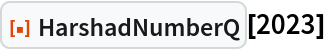Out=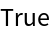Test another number:

 In:=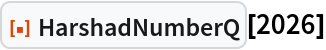Out=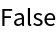### Scope (2)

Test a list of numbers:

 In:=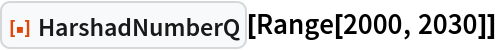Out=Give the base explicitly:

 In:=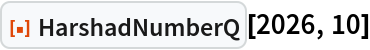Out=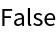Use a different base:

 In:=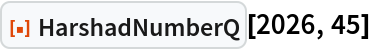Out=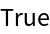### Possible Issues (3)

Base 0 can not be computed:

 In:=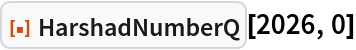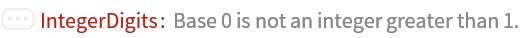Out=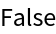Base 1 can not be computed:

 In:=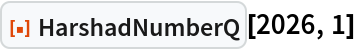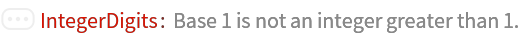Out=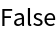The number 0 can not be divided by itself:

 In:=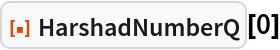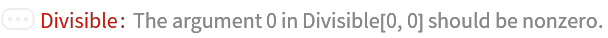Out=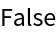### Neat Examples (4)

12 is a harshad number in multiple bases:

 In:=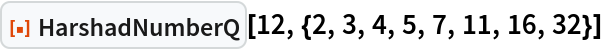Out=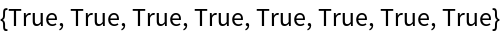12 is not an 8-harshad number:

 In:=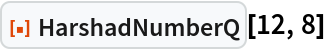Out=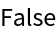The number 2016502858579884466176 is a multiple harshad number:

 In:=Out=In:=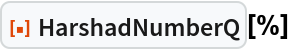Out=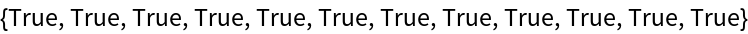Find the first 1000 harshad numbers and visualize them:

 In:=Out=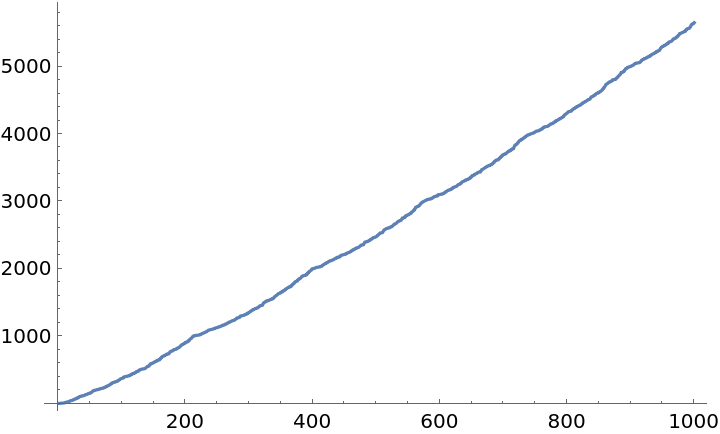All numbers up to a 10000 are either a harshad number, or the sum of two harshad numbers:

 In:=Out=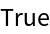SHuisman

## Version History

• 1.1.0 – 22 February 2023
• 1.0.0 – 09 January 2023